## 【建议收藏】16个OpenCV函数开始你的计算机视觉之旅_opencv常用函数_AIRX三次方的博客-程序员宝宝

1.我们如何清理图像数据集？图像有不同的形状和大小

2.数据获取中一直存在的问题。在建立计算机视觉模型之前，我们应该收集更多图像吗？

3.学习深度学习对建立计算机视觉模型是否必不可少？我们可以不使用机器学习技术吗？

4.我们可以在自己的机器上建立计算机vsiion模型吗？并非每个人都可以使用GPU和TPU！### 目录

1. 什么是计算机视觉？

2. 为什么要将OpenCV用于计算机视觉任务？

3. 读取，写入和显示图像

4. 改变色彩空间

5. 调整图像大小

6. 影像旋转

7. 图片翻译

8. 简单图像阈值

9. 自适应阈值

10. 图像分割（分水岭算法）

11. 按位运算

12. 边缘检测

13. 图像过滤

14. 影像轮廓

15. 尺度不变特征变换（SIFT）

16. 加速的强大功能（SURF）

17. 特征匹配

18. 人脸检测

### 什么是CV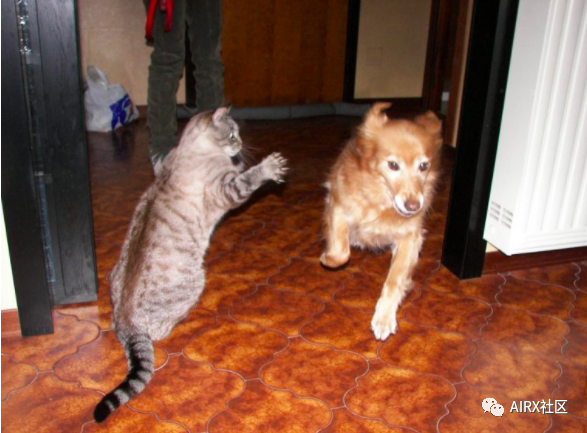### 关于OpenCV

OpenCV库最初是英特尔的一项研究项目。就其拥有的功能而言，它是目前最大的计算机视觉库。OpenCV包含2500多种算法的实现！它可免费用于商业和学术目的。该库具有适用于多种语言的接口，包括Python，Java和C ++。

OpenCV的第一个版本1.0于2006年发布，此后OpenCV社区发展迅猛。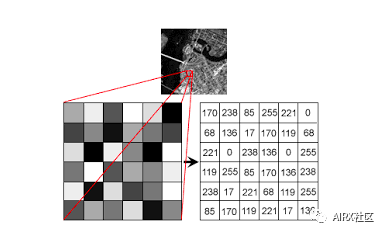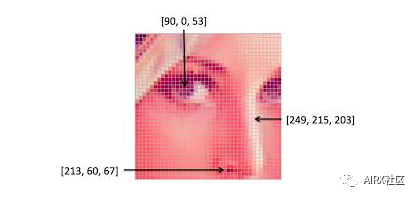``#import the libraries``import numpy as np``import matplotlib.pyplot as plt``import cv2``%matplotlib inline``#reading the image ``image = cv2.imread('index.png')``image = cv2.cvtColor(image,cv2.COLOR_BGR2RGB)``#plotting the image``plt.imshow(image)``#saving image``cv2.imwrite('test_write.jpg',image)``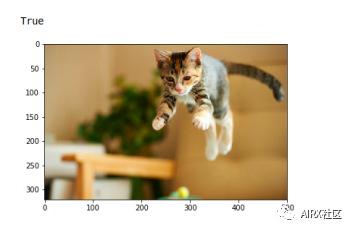### 改变色彩空间

``#import the required libraries ``import numpy as np ``import matplotlib.pyplot as plt ``import cv2 ``%matplotlib inline ``image = cv2.imread('index.jpg') ``#converting image to Gray scale ``gray_image = cv2.cvtColor(image,cv2.COLOR_BGR2GRAY)``#plotting the grayscale image``plt.imshow(gray_image) ``#converting image to HSV format``hsv_image = cv2.cvtColor(image,cv2.COLOR_BGR2HSV)``#plotting the HSV image``plt.imshow(hsv_image)``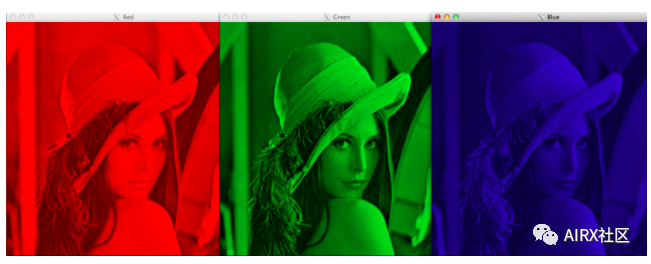### 调整图像大小

1.INTER_NEAREST：  最近邻居插值

2.INTER_LINEAR：  双线性插值

3.INTER_AREA：  使用像素面积关系进行重采样

4.INTER_CUBIC：  在4×4像素邻域上的双三次插值

5.INTER_LANCZOS4：在8×8邻域内进行Lanczos插值

OpenCV的调整大小功能默认情况下使用双线性插值。

``import cv2 ``import numpy as np ``import matplotlib.pyplot as plt ``%matplotlib inline ``#reading the image ``image = cv2.imread('index.jpg') ``#converting image to size (100,100,3) ``smaller_image = cv2.resize(image,(100,100),inerpolation='linear') ``#plot the resized image``plt.imshow(smaller_image)``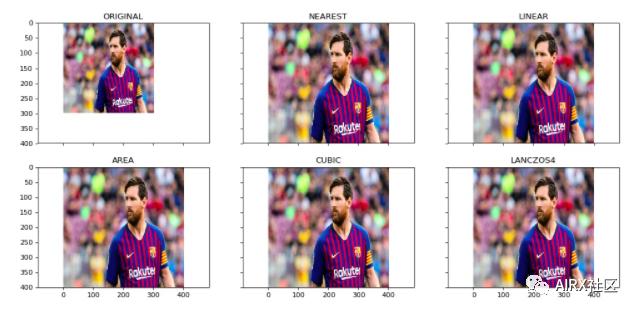### 影像旋转

“你需要大量数据来训练深度学习模型”。大多数深度学习算法在很大程度上取决于数据的质量和数量。但是，如果你没有足够大的数据集怎么办？并非所有人都能负担得起手动收集和标记图像的费用。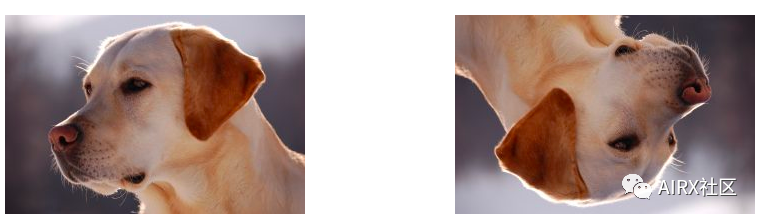``#importing the required libraries ``import numpy as np ``import cv2 ``import matplotlib.pyplot as plt ``%matplotlib inline ``image = cv2.imread('index.png') ``rows,cols = image.shape[:2] ``#(col/2,rows/2) is the center of rotation for the image ``# M is the cordinates of the center ``M = cv2.getRotationMatrix2D((cols/2,rows/2),90,1) ``dst = cv2.warpAffine(image,M,(cols,rows)) ``plt.imshow(dst)``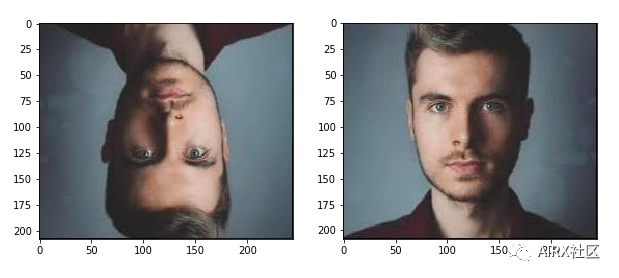### 图片翻译

X = x + dx

Y = y + dy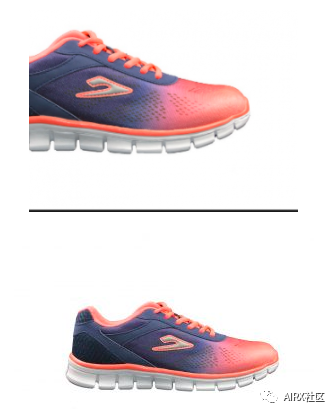``#importing the required libraries ``import numpy as np ``import cv2 ``import matplotlib.pyplot as plt ``%matplotlib inline ``#reading the image``image = cv2.imread('index.png')``#shifting the image 100 pixels in both dimensions``M = np.float32([[1,0,-100],[0,1,-100]]) ``dst = cv2.warpAffine(image,M,(cols,rows)) ``plt.imshow(dst)``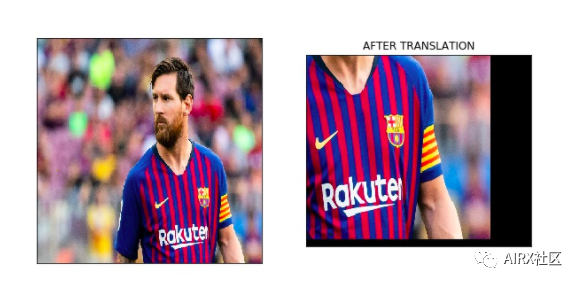### 简单图像阈值

````#importing the required libraries ``import numpy as np ``import cv2 ``import matplotlib.pyplot as plt ``%matplotlib inline `
`#here 0 means that the image is loaded in gray scale format``gray_image = cv2.imread('index.png',0)`
`ret,thresh_binary = cv2.threshold(gray_image,127,255,cv2.THRESH_BINARY)``ret,thresh_binary_inv = cv2.threshold(gray_image,127,255,cv2.THRESH_BINARY_INV)``ret,thresh_trunc = cv2.threshold(gray_image,127,255,cv2.THRESH_TRUNC)``ret,thresh_tozero = cv2.threshold(gray_image,127,255,cv2.THRESH_TOZERO)``ret,thresh_tozero_inv = cv2.threshold(gray_image,127,255,cv2.THRESH_TOZERO_INV)`
`#DISPLAYING THE DIFFERENT THRESHOLDING STYLES``names = ['Oiriginal Image','BINARY','THRESH_BINARY_INV','THRESH_TRUNC','THRESH_TOZERO','THRESH_TOZERO_INV']``images = gray_image,thresh_binary,thresh_binary_inv,thresh_trunc,thresh_tozero,thresh_tozero_inv`
`for i in range(6):``    plt.subplot(2,3,i+1),plt.imshow(images[i],'gray')``    plt.title(names[i])``    plt.xticks([]),plt.yticks([])`
`plt.show()````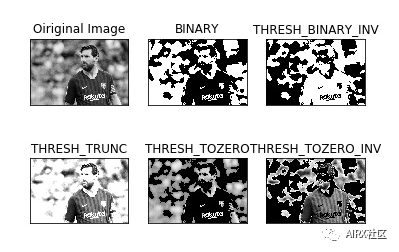### 自适应阈值

````#import the libraries``import numpy as np``import matplotlib.pyplot as plt``import cv2``%matplotlib inline`
`#ADAPTIVE THRESHOLDING``gray_image = cv2.imread('index.png',0)`
`ret,thresh_global = cv2.threshold(gray_image,127,255,cv2.THRESH_BINARY)``#here 11 is the pixel neighbourhood that is used to calculate the threshold value``thresh_mean = cv2.adaptiveThreshold(gray_image,255,cv2.ADAPTIVE_THRESH_MEAN_C,cv2.THRESH_BINARY,11,2)`
`thresh_gaussian = cv2.adaptiveThreshold(gray_image,255,cv2.ADAPTIVE_THRESH_GAUSSIAN_C,cv2.THRESH_BINARY,11,2)`
`names = ['Original Image','Global Thresholding','Adaptive Mean Threshold','Adaptive Gaussian Thresholding']``images = [gray_image,thresh_global,thresh_mean,thresh_gaussian]`
`for i in range(4):``    plt.subplot(2,2,i+1),plt.imshow(images[i],'gray')``    plt.title(names[i])``    plt.xticks([]),plt.yticks([])`
`plt.show()`
```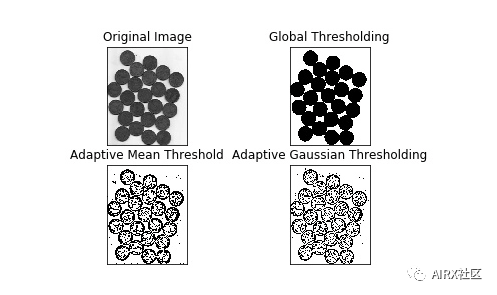### 图像分割（分水岭算法）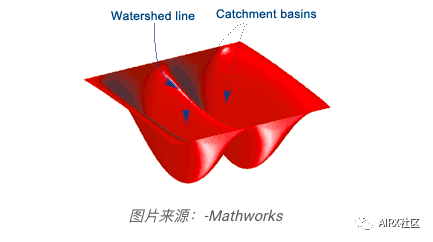````#importing required libraries``import numpy as np``import cv2``import matplotlib.pyplot as plt`
`#reading the image``image = cv2.imread('coins.jpg')``#converting image to grayscale format``gray = cv2.cvtColor(image,cv2.COLOR_BGR2GRAY)``#apply thresholding``ret,thresh = cv2.threshold(gray,0,255,cv2.THRESH_BINARY_INV+cv2.THRESH_OTSU)``#get a kernel``kernel = np.ones((3,3),np.uint8)``opening = cv2.morphologyEx(thresh,cv2.MORPH_OPEN,kernel,iterations = 2)``#extract the background from image``sure_bg = cv2.dilate(opening,kernel,iterations = 3)`
`dist_transform = cv2.distanceTransform(opening,cv2.DIST_L2,5)``ret,sure_fg = cv2.threshold(dist_transform,0.7*dist_transform.max(),255,0)`
`sure_fg = np.uint8(sure_fg)``unknown = cv2.subtract(sure_bg,sure_bg)`
`ret,markers = cv2.connectedComponents(sure_fg)`
`markers = markers+1`
`markers[unknown==255] = 0`
`markers = cv2.watershed(image,markers)``image[markers==-1] = [255,0,0]`
`plt.imshow(sure_fg)````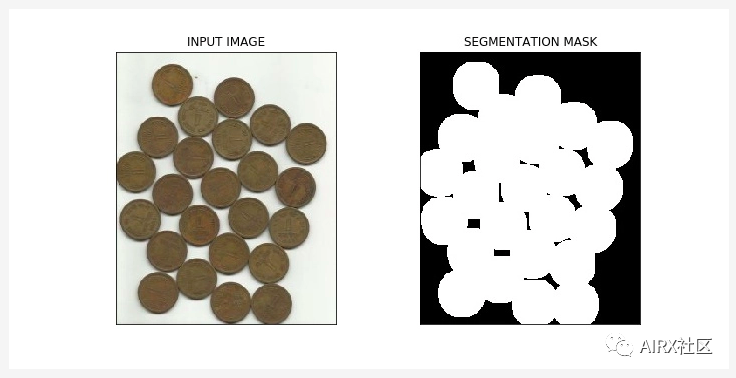### 换位运算

``#import required libraries``import numpy as np ``import matplotlib.pyplot as plt ``import cv2 ``%matplotlib inline ``#read the image``image = cv2.imread('coins.jpg')``#apply thresholdin ``ret,mask = cv2.threshold(sure_fg,0,255,cv2.THRESH_BINARY_INV+cv2.THRESH_OTSU) ``#apply AND operation on image and mask generated by thrresholding``final = cv2.bitwise_and(image,image,mask = mask) ``#plot the result``plt.imshow(final)``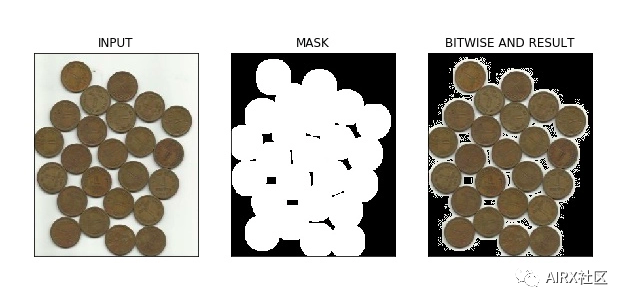### 边缘检测

• 深度不连续

• 表面取向不连续

• 材料特性的变化

• 场景照明的变化

````#import the required libraries``import numpy as np ``import cv2 ``import matplotlib.pyplot as plt ``%matplotlib inline``#read the image``image = cv2.imread('coins.jpg') ``#calculate the edges using Canny edge algorithm``edges = cv2.Canny(image,100,200) ``#plot the edges``plt.imshow(edges)`
```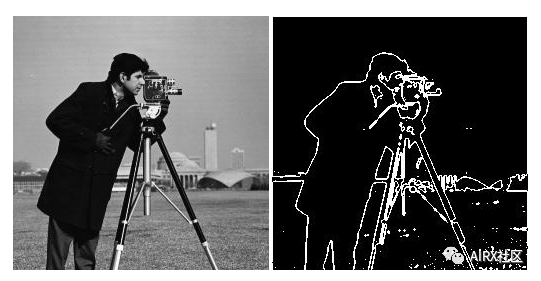### 图像过滤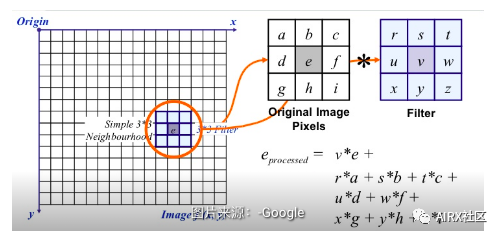````#importing the required libraries ``import numpy as np ``import cv2 ``import matplotlib.pyplot as plt ``%matplotlib inline ``image = cv2.imread('index.png') ``#using the averaging kernel for image smoothening ``averaging_kernel = np.ones((3,3),np.float32)/9 ``filtered_image = cv2.filter2D(image,-1,kernel) ``plt.imshow(dst) ``#get a one dimensional Gaussian Kernel ``gaussian_kernel_x = cv2.getGaussianKernel(5,1) ``gaussian_kernel_y = cv2.getGaussianKernel(5,1) ``#converting to two dimensional kernel using matrix multiplication ``gaussian_kernel = gaussian_kernel_x * gaussian_kernel_y.T ``#you can also use cv2.GaussianBLurring(image,(shape of kernel),standard deviation) instead of cv2.filter2D ``filtered_image = cv2.filter2D(image,-1,gaussian_kernel) ``plt.imshow()`
```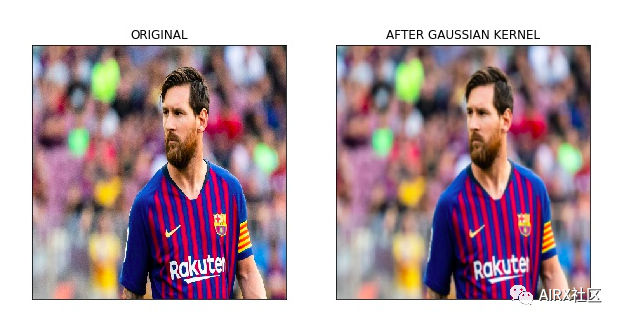### 影像轮廓

``#importing the required libraries ``import numpy as np ``import cv2 ``import matplotlib.pyplot as plt ``%matplotlib inline ``image = cv2.imread('shapes.png') ``#converting RGB image to Binary ``gray_image = cv2.cvtColor(image,cv2.COLOR_BGR2GRAY) ``ret,thresh = cv2.threshold(gray_image,127,255,0) ``#calculate the contours from binary image``im,contours,hierarchy = cv2.findContours(thresh,cv2.RETR_TREE,cv2.CHAIN_APPROX_SIMPLE) ``with_contours = cv2.drawContours(image,contours,-1,(0,255,0),3) ``plt.imshow(with_contours)``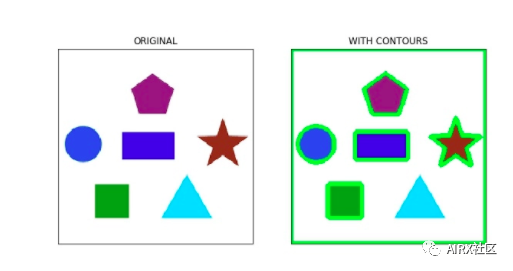### 尺度不变特征变换（SIFT）

• 尺度空间极值检测

• 关键点本地化

• 方向分配

• 关键点描述符

• 关键点匹配

````#import required libraries``import cv2``import numpy as np``import matplotlib.pyplot as plt``%matplotlib inline``#show OpenCV version``print(cv2.__version__)``#read the iamge and convert to grayscale``image = cv2.imread('index.png')``gray = cv2.cvtColor(image,cv2.COLOR_BGR2GRAY)``#create sift object``sift  = cv2.xfeatures2d.SIFT_create()``#calculate keypoints and their orientation``keypoints,descriptors = sift.detectAndCompute(gray,None)``#plot keypoints on the image``with_keypoints = cv2.drawKeypoints(gray,keypoints)``#plot the image``plt.imshow(with_keypoints)`
```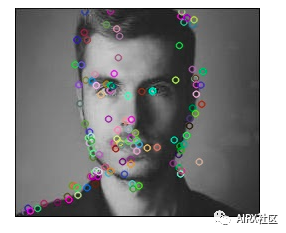### SURF

SURF是SIFT的增强版本。它的工作速度更快，并且对图像转换更健壮。在SIFT中，比例空间使用高斯的拉普拉斯算子近似。什么是高斯的拉普拉斯算子？

````#import required libraries``import cv2``import numpy as np``import matplotlib.pyplot as plt``%matplotlib inline``#show OpenCV version``print(cv2.__version__)``#read image and convert to grayscale``image = cv2.imread('index.png')``gray = cv2.cvtColor(image,cv2.COLOR_BGR2GRAY)``#instantiate surf object``surf  = cv2.xfeatures2d.SURF_create(400)``#calculate keypoints and their orientation``keypoints,descriptors = surf.detectAndCompute(gray,None)`
`with_keypoints = cv2.drawKeypoints(gray,keypoints)`
`plt.imshow(with_keypoints)````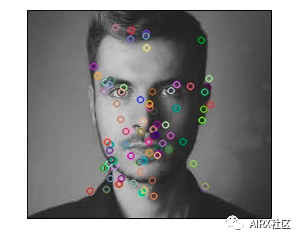### 特征匹配

````import numpy as np``import cv2``import matplotlib.pyplot as plt``%matplotlib inline`
`#reading images in grayscale format``image1 = cv2.imread('messi.jpg',0)``image2 = cv2.imread('team.jpg',0)`
`#finding out the keypoints and their descriptors``keypoints1,descriptors1 = cv2.detectAndCompute(image1,None)``keypoints2,descriptors2 = cv2.detectAndCompute(image2,None)`
`#matching the descriptors from both the images ``bf = cv2.BFMatcher()``matches = bf.knnMatch(ds1,ds2,k = 2)`

`#selecting only the good features``good_matches = []``for m,n in matches:``    if m.distance < 0.75*n.distance:``        good.append([m])`
`image3 = cv2.drawMatchesKnn(image1,kp1,image2,kp2,good,flags = 2)`
```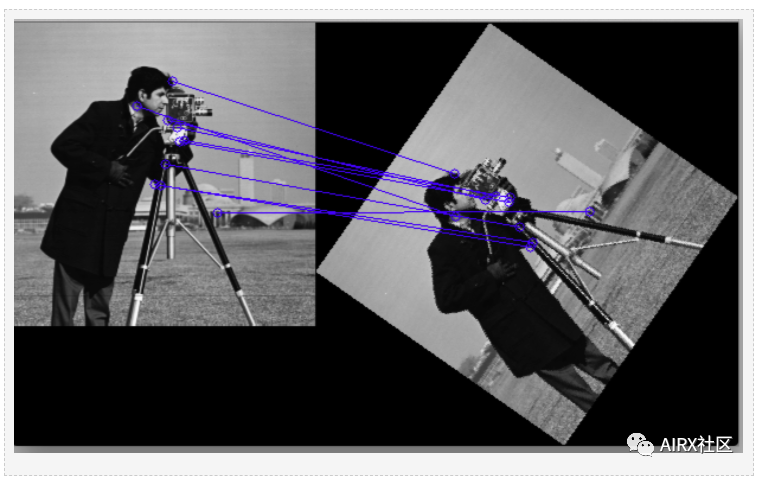### 人脸检测

OpenCV支持基于haar级联的对象检测。Haar级联是基于机器学习的分类器，可计算图像中的不同特征（如边缘，线条等）。然后，这些分类器使用多个正样本和负样本进行训练。

OpenCV Github存储库中提供了针对不同对象（如面部，眼睛等）的训练分类器，你还可以针对任何对象训练自己的haar级联。​​​​​​​

````#import required libraries``import numpy as np``import cv2 as cv``import matplotlib.pyplot as plt``%matplotlib inline`
`#load the classifiers downloaded ``face_cascade = cv.CascadeClassifier('haarcascade_frontalface_default.xml')``eye_cascade = cv.CascadeClassifier('haarcascade_eye.xml')``#read the image and convert to grayscale format``img = cv.imread('rotated_face.jpg')``gray = cv.cvtColor(img, cv.COLOR_BGR2GRAY)``#calculate coordinates ``faces = face_cascade.detectMultiScale(gray, 1.1, 4)``for (x,y,w,h) in faces:``    cv.rectangle(img,(x,y),(x+w,y+h),(255,0,0),2)``    roi_gray = gray[y:y+h, x:x+w]``    roi_color = img[y:y+h, x:x+w]``    eyes = eye_cascade.detectMultiScale(roi_gray)``    #draw bounding boxes around detected features``    for (ex,ey,ew,eh) in eyes:``        cv.rectangle(roi_color,(ex,ey),(ex+ew,ey+eh),(0,255,0),2)``#plot the image``plt.imshow(img)``#write image ``cv2.imwrite('face_detection.jpg',img)````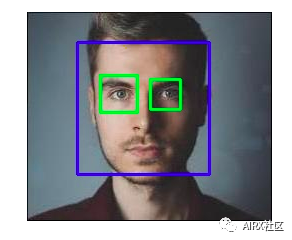### 光纤通信工程-光纤介绍（一）_光纤工程_Zhang-Zhen的博客-程序员宝宝

1、光纤的结构2、光传输分类3、光纤分类4、光纤的损耗吸收损耗散射损耗附加损耗5、三个低损耗窗口1310nm窗口1550nm窗口多模光纤用850nm窗口6、光纤衰减系数a = 10 lg（Pi/Po）/ LPi 为输入光功率Po为输出光功率L为光纤的长度7、光纤损耗测试方法剪短法插入法向后散射法...

### 158.9. FAQ_weixin_34292959的博客-程序员宝宝

158.9.1.err: Could not request certificate: No route to host - connect(2)err: Could not request certificate: Connection refused - connect(2)Exiting; failed to retrieve...